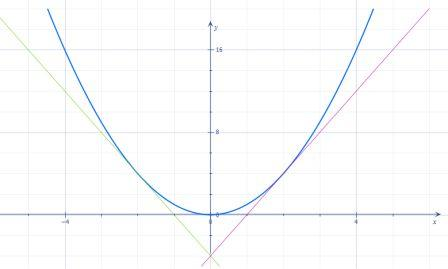# Draw a diagram to show that there are two tangent lines to the parabola y = x^2 that pass through...

## Question:

Draw a diagram to show that there are two tangent lines to the parabola {eq}y = x^2 {/eq} that pass through the point (0, - 4). Find the coordinates of the points where these tangent lines intersect the parabola.

## Tangent Lines

This problem is based on the application of the concept of tangent, slope, and parabola. We are going to start off by finding the slope of the desired tangents. Once we have the slope we will calculate the coordinates.

We have,

{eq}\displaystyle y=x^2 {/eq}

So, the point lying on the parabola will be of the form {eq}(x,x^2) {/eq}.

Let us assume that the tangent at the point {eq}(x,x^2) {/eq} passes through {eq}(0,-4) {/eq}.

The slope of the tangent will be,

{eq}\displaystyle \frac{dy}{dx}=2x {/eq}

So, the slope at the point {eq}(x,x^2) {/eq} will be, {eq}\displaystyle \frac{dy}{dx}=2x {/eq}.

Now, we are going to use two point formula, the tangent passes through {eq}(0,-4) {/eq} and {eq}(x,x^2) {/eq}.

So, the slope will be:

{eq}\displaystyle m=\frac{x^2-(-4)}{x-0}=\frac{x^2+4}{x} {/eq}

Equating both the slopes, we get,

{eq}\displaystyle \frac{x^2+4}{x}=2x {/eq}

{eq}\displaystyle x^2-4=0 {/eq}

{eq}\displaystyle x=\pm2 {/eq}

Thus, the required coordinates are:

{eq}\displaystyle \boxed{\displaystyle (2,4)} {/eq}

And

{eq}\displaystyle \boxed{\displaystyle (-2,4)} {/eq}

The sketch below shows both the tangents and the parabola: